# PSAT Math : How to find the amount of sales tax

## Example Questions

### Example Question #1 : How To Find The Amount Of Sales Tax

If there is a 10% sale on an item, and then 9% sales tax is applied to that after-sale price, then what is the total cost of the item including tax as a percentage of its pre-sale sticker price?

99.2%

81.9%

99%

98.1%

101%

98.1%

Explanation:

A 10% sale means that the post-sale price of the item is now 90%, or 0.9 of the original cost of the item. We then apply 9% sales tax by multiplying the 0.9 by 109%, or 1.09. 0.9 * 1.09 = .981, so the total cost of the item is 98.1% of the original pre-sale sticker price.

For percentage problems that do not deal with a specific starting number, it is always helpful to plug in 100 for the starting number. Here, we would then have a post-sale price of 90 dollars, and if we calculate the sales tax for the 90-dollar item it would be 90 * 0.09 = $8.10. THis gives us a total cost of 90 + 8.10 =$98.10, or 98.1% of the original 100-dollar price.

### Example Question #2 : How To Find The Amount Of Sales Tax

Cynthia buys 2 shirts, each costing $35, a pair of pants for$40 and a belt for $18. At the register the total she owes is$138.24.  What is the rate of sales tax in her state?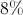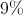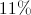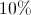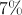Explanation:

To find the amount of sales tax, take the difference in the total before and after tax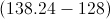and divide by the price before tax.  This gives 0.08 or 8%.

A certain state charges sales tax at a rate of 8.75% per dollar spent. John spends $127.50 total, including tax, on clothes. What was the total price of the clothing before tax was added? Possible Answers: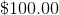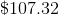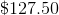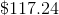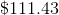Correct answer:Explanation: Since we know the total price with tax, we simply need to work backwards to remove the tax from the total price and find the price of the clothing itself. This can be done as follows: Clothing Price or C = Total price / 1 + tax rate C =$127.50/(1 + 0.0875) = $117.24 ### Example Question #4 : How To Find The Amount Of Sales Tax Jack is at the grocery store. He buys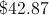in groceries, and groceries are not taxed in his state. He also buys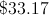in other items, which are taxed at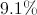(not yet applied). How much is his total? Possible Answers: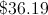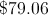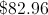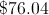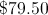Correct answer:Explanation: Jack's groceries are not taxed, so they cost, but his other items are taxed. We will calculate the price with the tax: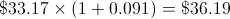We will add the two together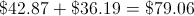### Example Question #5 : How To Find The Amount Of Sales Tax Harry, on his first lunch date with Sally, decides to put the entire bill on his credit card. If the bill came to$28 and Harry wants to leave an 18% tip, what is the total amount that he should pay?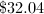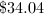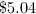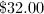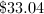Explanation:

To find an 18% increase, multiply by 1.18.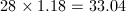Lisa just bought a desktop computer. The computer cost $1500, the keyboard cost$100, and the mouse cost $25. If the local sales tax is 7%, what was the total cost of her purchases? Possible Answers: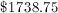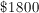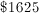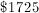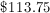Correct answer:Explanation: Total Tax =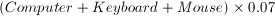=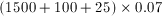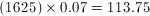Total Cost = Computer + Keyboard + Mouse + Tax =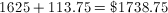### Example Question #7 : How To Find The Amount Of Sales Tax Mike spent$30 on food and $50 on non-food products in the supermarket. There's a sales tax of 10% on non-food products only. How much did Mike spend in total at the supermarket? Possible Answers: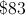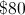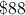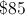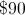Correct answer:Explanation: Since sales tax is only applicable to non-food products only, we can multiply 50 by 1.1 to get the total price of non-food products. In this case, it is$55. Add this value to the $30 spent on food for a total of$85.